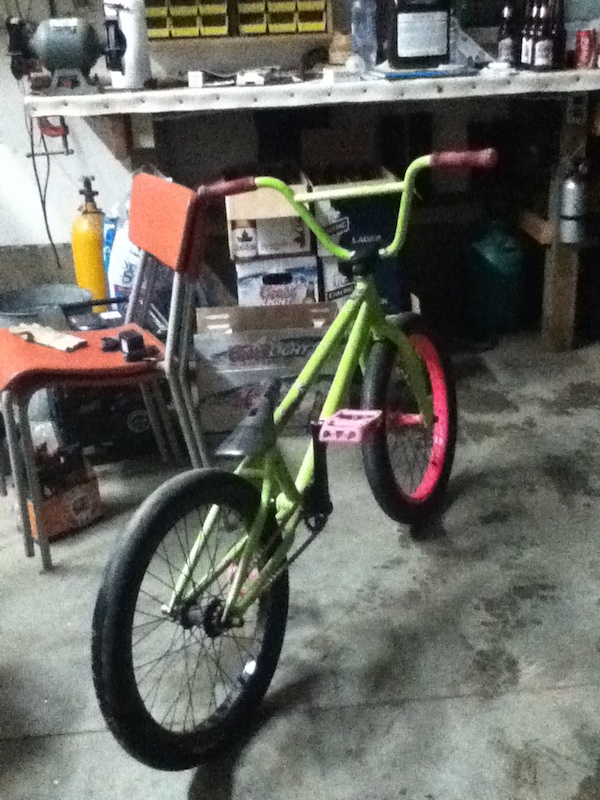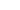• + 2Sundayftw (Feb 25, 2011 at 22:13)
Will you part out the pink rim and hub?
• + 3julienbmx779 (May 23, 2011 at 14:40)
gay
• + 1hubble (Jul 4, 2011 at 20:12)
ARE U GAY u say it on every pick
• + 1Nick150 (Sep 19, 2011 at 4:53)
ILL GIVE YOU MY DIRT JUMPER WITH 3 EXTRA GRIPS!!! MY BIKE IS \$800 BRAND NEW
• + 1HeWhoIsNotTuba5 (Jan 12, 2011 at 19:02)
sweet ride how's the frame holding up?
• + 1jonathanpbmx (Sep 11, 2011 at 18:34)
600 for it with the pink rime
• + 1bmxr-369 (Apr 29, 2011 at 8:28)
• + 1wyomingbiker (Jun 6, 2012 at 7:29)
was that Nicky Beattys old bike
• + 1jonathanpbmx (Jul 16, 2011 at 16:55)
trade 4 mirraco phelm and 300
• + 1HeWhoIsNotTuba5 (Jan 14, 2011 at 13:23)
I'm suprised ha I broke mine
• + 1jonathanpbmx (Jul 15, 2011 at 15:16)
i will trade u my 2011 mirraco phelm
• + 1sundayfundayam2010 (Aug 10, 2011 at 18:16)
how much for front wheel
• + 1littlehewy (Mar 8, 2012 at 20:07)
Is this still for sale?
• + 1Pjvitali (Jul 30, 2013 at 20:04)
Wat kind of front rim?
• + 1riheem13 (Oct 29, 2012 at 0:04)
Eastern is F*cking gay
• + 1radar123 (Aug 6, 2011 at 5:46)
nope
• + 1radar123 (Jul 15, 2011 at 16:18)
no thanks
• + 1radar123 (Feb 26, 2011 at 6:45)
nope sorry
• + 0radar123 (Jan 13, 2011 at 5:19)
thanks and the frame hholds up fine
• + 1VerdeRide-R180 (Jan 12, 2011 at 19:08)
pree sickk man
• + 1radar123 (Apr 29, 2011 at 15:44)
how much cash ?
• + 1radar123 (May 23, 2011 at 15:40)
jelous
• + 1radar123 (Jul 16, 2011 at 19:31)
nope
• + 1radar123 (Jul 15, 2011 at 16:18)
no thanks
• + 1bmxboyD (Jul 6, 2011 at 22:55)watup (Aug 5, 2011 at 20:03)Bikerider250 (Dec 22, 2011 at 17:16)